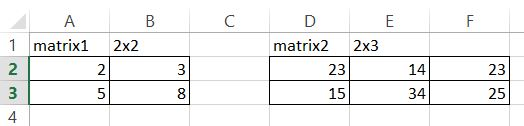### Matrix Multiplication In Excel

In this Excel tutorial you will teach yourself about matrix multiplication in Excel. You will see how to use MMULT Excel function to multiply matrixes.

First you need matrixes. Here are my sample matrixes.Then you need to use MMULT Excel function to multiply them.

MMULT function takes matrixes as arguments so it is obvious to use.

To use it highlight the whole matrix and type formula in first cell.

MMULT formula in my example is

=MMULT(A2:B3,D2:F3)This is an array formula so press CTRL + SHIFT + ENTER keyboard shortcut.This is how multiplied matrix looks like.

## Template

```Further reading: